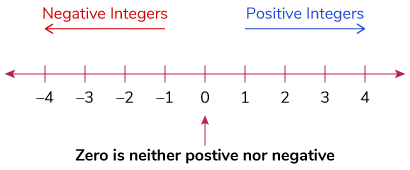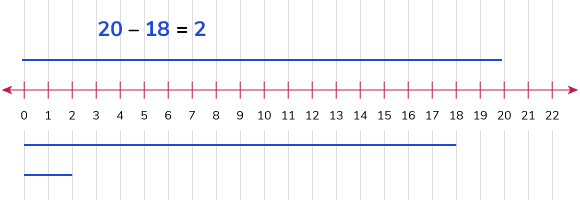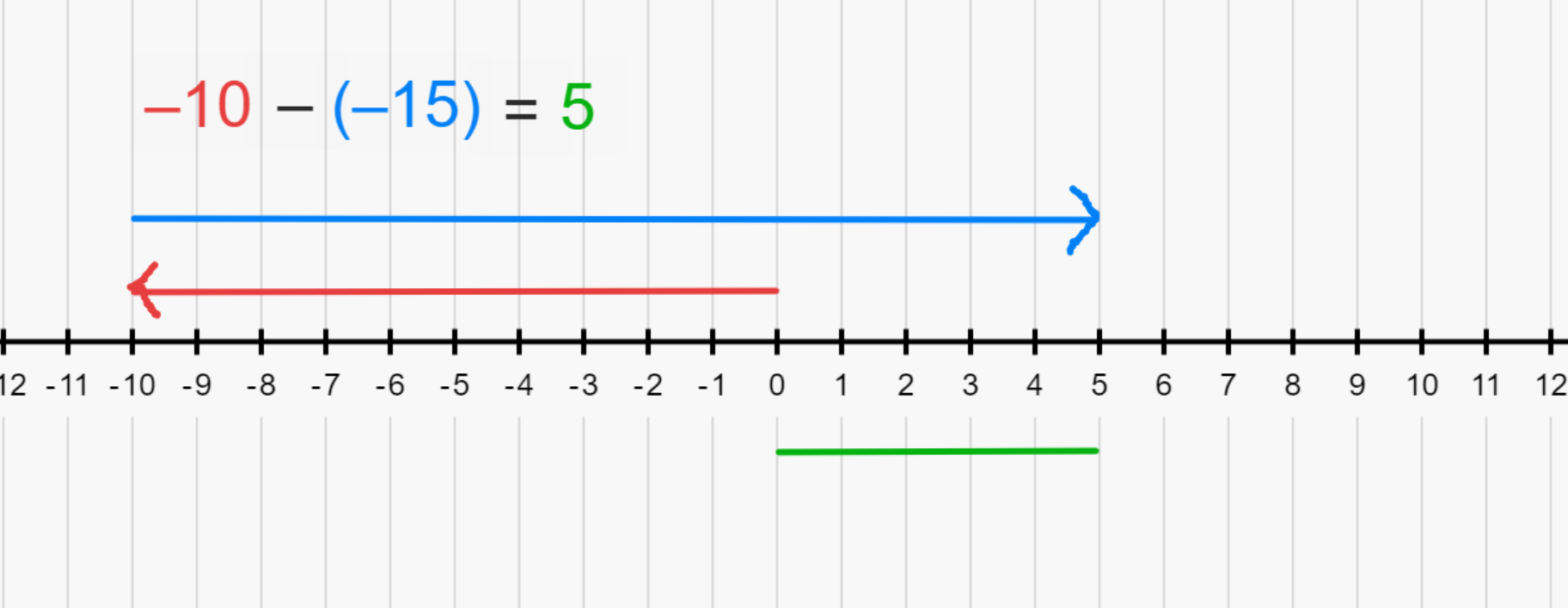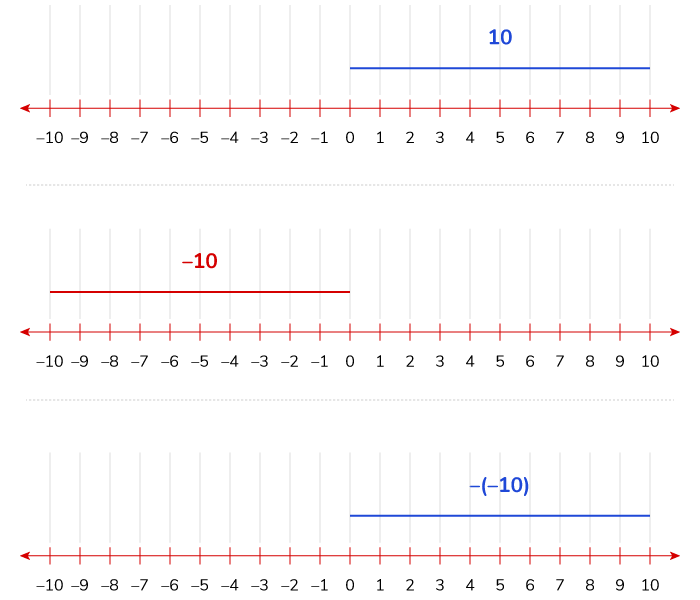# Addition and Subtraction of Integers

Go back to  'Integers'

## Introduction to Addition and Subtraction of Integers

If you are comfortable with visualising the addition and subtraction of whole numbers on the number line, the same concept can be easily extended to integers.

## The idea behind Addition and Subtraction of Integers

Numbers to the right are positive numbers, numbers to the left are negative numbers, and zero is neither positive nor negative.
As understood, the basic principles of addition are repeatedly adding into a particular group and subtracting is nothing but removing from a group. Operations on integers work similarly, but with a twist1. If both numbers have the same sign, add both numbers and include the same sign as both.

Example:

1. 4 + 8 = 12
Using the number line, we start at zero and move to 4. The equation calls for adding 8 to 4. From 4, move 8 steps towards the right of the number line, leaving us at 12.
2. –7 + (–10) = –17
Using the number line, we begin at 0 and move to the left and stop at –7. Since the equation calls for adding -10 to -7, move another 10 steps towards the left, leaving us at –17.

2. If both numbers have different signs, add or subtract the number and include the sign of the largest absolute integer.

Example:

1. 10 + (– 4)
a. 10 – 4 = 6
We begin at 0, moving to 10 spaces to the right of the number line. The equation then asks you to add a –4. But a negative sign means you have to travel to the left of the number line, making you move 4 spaces to the left from 10. Landing you at 6.
2. –8 + –9
a. –8 – 9 = –17
You begin at –8 on the number line and move 9 spaces to the left as you have to add –9 to –8. You will land at –17.

### Rules for Subtracting Integers

1. If both numbers have positive signs, the difference will be positive.

Example:

20 – 18 = 2
Starting at 0, move 20 spaces to the right of the number line. As you have to subtract 18, move 18 spaces to the left of the number line. Landing at 2.2. If both numbers have a negative sign, subtract the two numbers and include the sign of the largest absolute value.

Example:

–10 – ( –15)
Starting at 0, move to the left of the number line at –10. Since two negatives make a positive, making – (–15) = 15, we move forward towards the right of the number line. From –10 we move 15 spaces to the right, landing at 5.3. If both numbers have different signs, subtract the two and denote the difference with the sign of the largest absolute value.

Example:

1. 18 – 5 = 13
18 is larger than 5 and has a positive value
.

## Fun Facts about Integers and Signs

One thing that children struggle with it is signs that precede integers, specifically the negative sign. Here is a very simple algorithm to help visualise and internalise the sign changes and how that affects the direction of a number on the number line:
Let’s the number 10 for our particular example (keep in mind this rule will apply to all integers):As you can see, with the addition of each negative sign the direction of the line flips. That is when we have 10, the number line representation is towards the right that is positive number, when it’s –10, it switches to the left that is negative number, again when another negative sign is added, –(–10), we get back the positive number, 10 and the number line representation switches to the right.

Learn from the best math teachers and top your exams

• Live one on one classroom and doubt clearing
• Practice worksheets in and after class for conceptual clarity
• Personalized curriculum to keep up with school

0